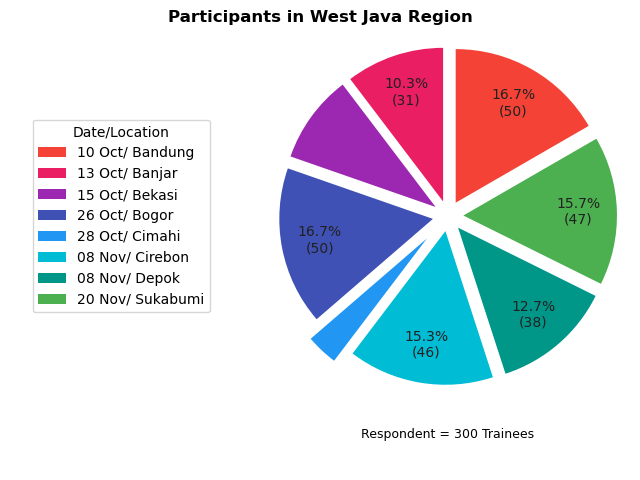# Matplotlib - Pie Chart - Part Three

Python Visualization Article Series

This is a multiple-parts article. There are few sections here.

Table of Content
Where to Discuss?

Local Group

### Preface

Goal: Conclude the pie chart view.

Let’s make things complex.

### 1: Modularizing

Before we begin, we need to separate reusable code, into different script.

• `colors.py`
• `helper.py`
• `trainees.py`

So we don’t have to retype all the time.

#### Colors

``````# dict { color name: rgb code }

material_colors = {
'red500'   : '#F44336',
'pink500'  : '#E91E63',
'purple500': '#9C27B0',
'indigo500': '#3F51B5',
'blue500'  : '#2196F3',
'cyan500'  : '#00BCD4',
'teal500'  : '#009688',
'green500' : '#4CAF50',
'lime500'  : '#CDDC39',
'orange500': '#FF9800'
}

colors = list(material_colors.values())``````

#### Helper

``````# additional function

def wedge_text(percent, total):
absolute = int(round(total*percent/100))
if percent > 10:
return "{:.1f}%\n({:d})".format(percent, absolute)
else:
return ""``````

#### Trainees

``````# dict { location : (trainee, date, additional data) }

response = {
'Bandung' : (50, '10 Oct', 0.1),
'Banjar'  : (31, '13 Oct', 0.1),
'Bekasi'  : (28, '15 Oct', 0.1),
'Bogor'   : (50, '26 Oct', 0.1),
'Cimahi'  : (10, '28 Oct', 0.2),
'Cirebon' : (46, '08 Nov', 0.1),
'Depok'   : (38, '08 Nov', 0.1),
'Sukabumi': (47, '20 Nov', 0.1)
}

elements = list(response.values())
trainees = [el for el in elements]
date     = [el for el in elements]
explode  = [el for el in elements]
legends  = [
"%s/ %s" % (value, key)
for key, value
in response.items() ]
total    = sum(trainees)``````

### 2: Data Test Bed

#### Combine All

Consider combine all the script above

``````import numpy as np
import matplotlib.pyplot as plt

from colors import *
from trainees import *
from helper import wedge_text``````
• Beware that we have a longer legend text.

#### Matplotlib

The chart code, is very similar with the previous one. With little differences.

``````# plot pie chart
axes = plt.subplot()

wedges, texts, autotexts = axes.pie(
trainees,
labels  = None,
colors  = colors,
explode = explode,
autopct = lambda percent: wedge_text(percent, total))

axes.legend(
wedges, legends,
title="Date/Location",
loc="center left",
bbox_to_anchor=(-0.6, 0.5, 0, 0))

axes.set_position([0.4, 0, 0.6, 1])

plt.show()``````

Wwe have a longer legend text. So we need to create more space

``axes.set_position([0.4, 0, 0.6, 1])``

Since we use `bbox_to_anchor` setting. the setting should also be changed.

``  bbox_to_anchor=(-0.6, 0.5, 0, 0)``

#### Chart Result

The chart result is drawn as expected. It is shown in figure below:### 3: Chart Decoration

We can add more chart decoration such as `title`, `suptitle`, and also a few enhancement.

#### Title and Suptitle

This line below is self explanatory.

``````plt.suptitle("Participants in West Java Region",
horizontalalignment='center',
weight="bold",
fontsize=12)
plt.title(   "Respondent = %d Trainees" % total,
horizontalalignment='center',
fontsize=9, y=-0.1)

plt.show()``````

#### Positioning

Since we add more object, we need more whitespace. So we should set the chart positioning again.

``````axes.legend(
wedges, legends,
title="Date/Location",
loc="center left",
bbox_to_anchor=(-0.6, 0.5, 0, 0))

axes.set_position([0.4, 0.1, 0.6, 0.9])``````

#### More Parameters

For learning and curiosity purpose, I add more paramater:

``````wedges, texts, autotexts = axes.pie(
trainees,
labels  = None,
colors  = colors,
explode = explode,
startangle=30,
pctdistance=0.75,
textprops=dict(color="#212121"),
autopct = lambda percent: wedge_text(percent, total))``````

#### Chart Result

Now we can see the result:All done properly.

### 4: Fancy Piechart

We can go further with visual.

#### Original Article

I found this amazing article, by `Kevin Amipara`.

We need to achieve the same effect.

#### Subplots instead of just subplot.

Wait…! Is this a plural form?

Since we need to draw object in figure, we need to change a little bit from:

``````# plot pie chart
axes = plt.subplot()``````

Into this form:

``````# plot pie chart
fig, axes = plt.subplots(figsize=(8, 5))``````

With this form we can make multiple chart. But in this case. Just drawing a circle.

#### Drawing Circle

The code is almost verbatim copy carbon, taken from the original article.

``````#draw circle
centre_circle = plt.Circle((0,0),0.80,fc='white')
fig = plt.gcf()

Instead of explaing the code here. You’d better read the original article.

We need to make a few adjustment as usual, so we can have proper chart.

``````wedges, texts, autotexts = axes.pie(
trainees,
labels  = None,
colors  = colors,
explode = explode,
startangle=30,
pctdistance=0.5,
textprops=dict(color="#212121"),
autopct = lambda percent: wedge_text(percent, total))

axes.legend(
wedges, legends,
title="Date/Location",
loc="center left",
bbox_to_anchor=(-0.6, 0.5, 0, 0))

axes.set_position([0.4, 0.1, 0.6, 0.8])``````

#### Chart Result

Finally, the fancy chart.:This works require creativity. And of course, imagination.

### What is Next 🤔?

After pretty pie chart, we can continue to cumulative bar chart.

Consider continue reading [ Matplotlib - Bar Chart - Part One ].